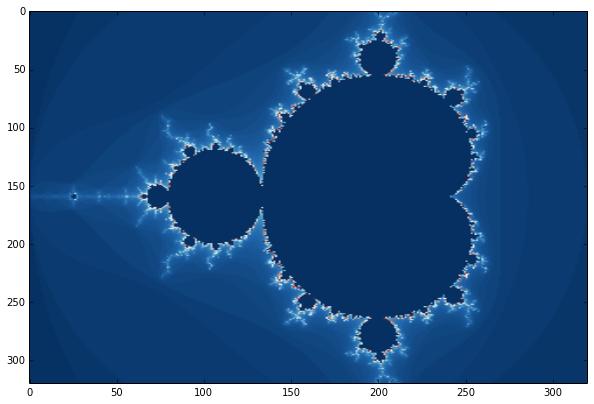# Mandelbrot Sets¶

#### Imports¶

In :
import matplotlib.pyplot as plt
import numpy as np
from pylab import cm

%matplotlib inline


#### Settings¶

In :
# Establish the area of the plot to look at. The
# Mandelbrot set is a fractal defined to exist in
# the set of imaginary numbers.
#
# You can see the whole set when you "zoom out" so that:
#
#     real axes go from  -2 to 1
#     imaginary axes from -1 to 1
#
# For this notebook the view is defined by two points. The
# "upper left" and the "lower right". To see the whole set
# you would use an upper of -2 + 1j and a lower of -1 + 1j.

# The view is defined by two complex numbers.
g_view = {"upper": -2 + 1j, "lower": 1 - 1j}
#g_view = {"upper": 0+0j, "lower": 1 - 1j}

# The resolution of the image in pixels
# The larger the image, the more time it will take to calculate
g_resolution = 320

# The maximum iterations. The more iterations, the longer it will
# take to calculate the mandelbrot set.
g_max_iterations = 128


#### Functions¶

In :
def complex_range(upper, lower, resolution):
"""Yields an iterable range of pixel points and complex numbers"""
step_y = (upper.imag - lower.imag) / (resolution - 1)
step_x = (lower.real - upper.real) / (resolution - 1)
for x in range(resolution):
real = upper.real + (x * step_x)
for y in range(resolution):
yield x, y, complex(real, lower.imag + (y * step_y))

In :
def test_point(point, max_iterations):
"""Test a point for inclusion in Mandelbrot set.
1.0 means it is in the set. Anything else is out."""
zn = complex(0,0)
c = point
for n in range(1, max_iterations + 1):
zn = zn * zn + c
if abs(zn) >= 2:
return 1 - (float(n) / max_iterations)
return 1.0

In :
def aspect_ratio(upper, lower):
return (upper.imag - lower.imag) / (lower.real - upper.real)


#### Generate an Image¶

In :
# Calculate the mandelbrot
i = np.array([*g_resolution]*g_resolution, dtype=float)
for x, y, c in complex_range(g_view["upper"], g_view["lower"], g_resolution):
i[y][x] = test_point(c, g_max_iterations)

In :
# Show the mandelbrot
plt.figure(figsize=(10,10))
#plt.axis([g_view["upper"].real, g_view["lower"].real, g_view["lower"].imag, g_view["upper"].imag])
img = plt.imshow(i, aspect=aspect_ratio(g_view["upper"], g_view["lower"])) #, cmap=cm.gray)

# Try different colormaps
#img.set_cmap('Greys')
img.set_cmap('RdBu')
#img.set_cmap('gray')
#img.set_cmap('binary')
#img.set_cmap('jet')
#img.set_cmap('gist_rainbow')
#img.set_cmap('hot')
#img.set_cmap('afmhot')
#img.set_cmap('RdGy')
#img.set_cmap('RdYlGn')
#img.set_cmap('RdYlBu')
#img.set_cmap('Dark2')
#img.set_cmap('hsv')

#plt.colorbar()
#img.write_png("mandelbrot.png")# Resources¶